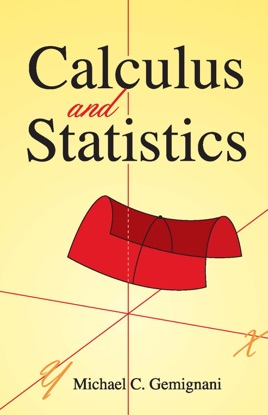• 11,99 €

## Descrizione dell’editore

Self-contained and suitable for undergraduate students, this text offers a working knowledge of calculus and statistics. It assumes only a familiarity with basic analytic geometry, presenting a coordinated study that develops the interrelationships between calculus, probability, and statistics.
Starting with the basic concepts of function and probability, the text addresses some specific probabilities and proceeds to surveys of random variables and graphs, the derivative, applications of the derivative, sequences and series, and integration. Additional topics include the integral and continuous variates, some basic discrete distributions, as well as other important distributions, hypothesis testing, functions of several variables, and regression and correlation. The text concludes with an appendix, answers to selected exercises, a general index, and an index of symbols.